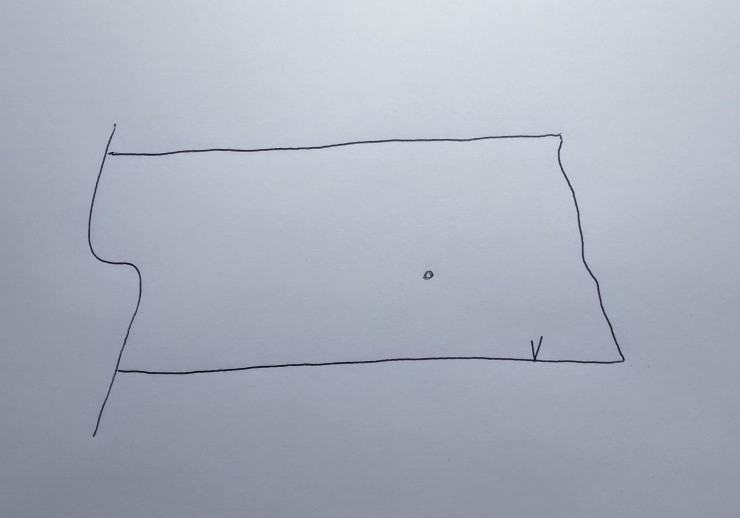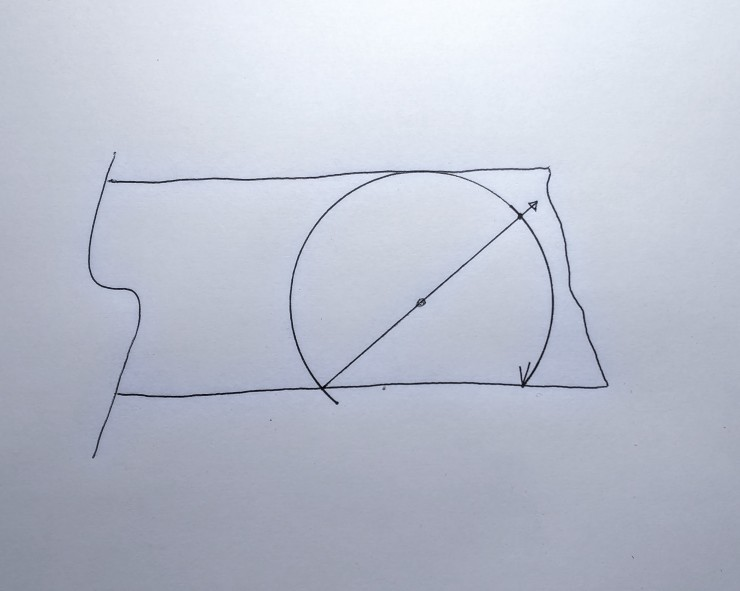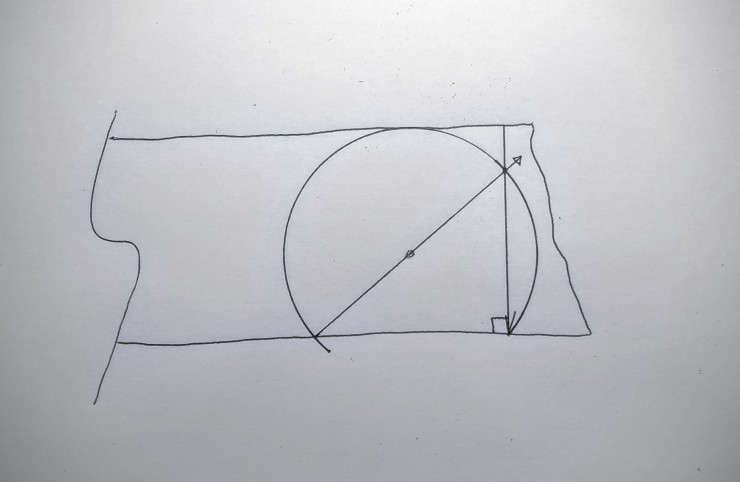The following sequence of steps show how to use simple geometry to create a right angle along the edge of a board–demonstrating that you don’t need a square to make a square layout line. Handy if you are making a square clamping jig to clamp the blade and tongue of a square together so you never have to do this again! The resulting try square is essentially a template of a right angle.Put a mark on the edge of the board where you want to construct the right angle crosscut.Locate the focal point of a circle that will pass through the starting mark. The circle must be sized to fit on the board as shown above.Draw a diameter line as shown from the edge of the board intersection through the focal point.Draw a line from the V mark through the point where the diameter line passes through the rim of the circle. This line will be square to the boards edge as predicted by Thales theorem.